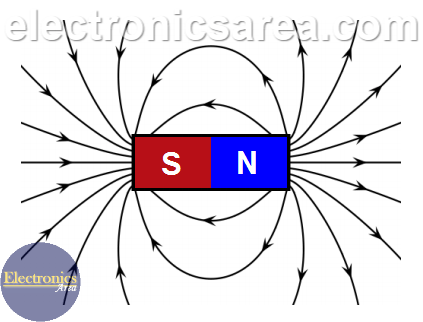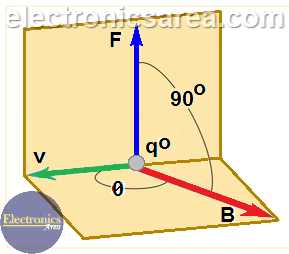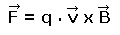Magnetic Field – Magnetic Field Lines

Magnetic Field Definition

1 – Magnets have a characteristic force that is called magnetic force.
2 – Moving charges such as electric current behave like magnets.

In the first case (1), the magnetic force and the field are a consequence of the existence of the magnetic poles (North and South poles). The magnetic field lines allow us to make an approximate estimate of the existing magnetic field at a given point, taking into account the following characteristics:

• The field lines are always closed loops that go from the North pole to the South pole outside the magnet and from the South pole to the North pole inside the magnet.
• Magnetic loops never crisscross.
• The magnetic lines of different magnets attract and repel each other: The lines of the same direction are attracted and the lines of the opposite direction repel each other.
• In the second case (2), the effect of magnetism is due to a flow of electrons (electric current) as in the case of a magnet and is independent of the medium.The Magnetic Field Vector

See the following diagram:Experimentally, when a charge “qo” with a velocity “v” approaches a magnet or a wire through which an electric current flows, a magnetic force “F” appears on the charge “qo”. The magnetic force depends on the magnetic field and the value and the direction of the speed of the charge. See the following formula:In the formula “F”, “v” and “B” are vectors, and “q0” is an scalar number. The magnetic force F is a vector obtained by the multiplication of the vector “v” (speed) and the vector “B” (magnetic field), and … the result is multiplied by “q” (scalar multiplication).

The direction of the resultant magnetic force “F” is perpendicular to both, the speed vector “v” and the magnetic field vector “B”.

• 1
•
• 4
•
•
•
•
•
•
5
Shares
•
5
Shares
• 1
•
• 4
•
•
•
•
•